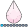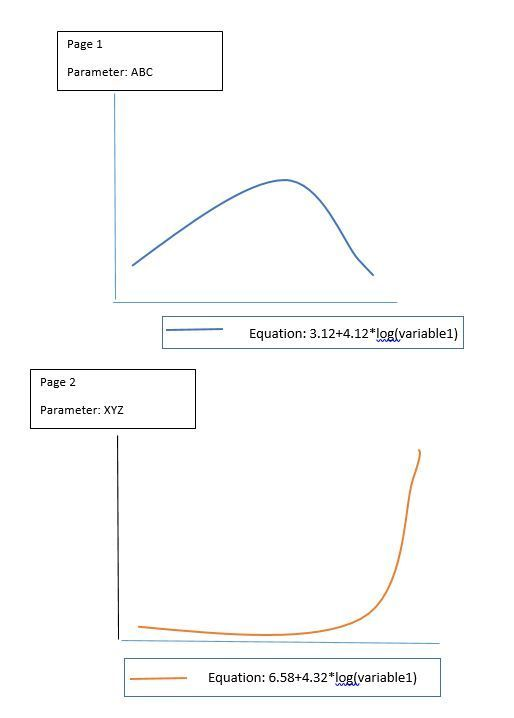## Multiple legends PROC SGPLOT

Hi Everyone,

I am creating a series plot/graph using PROC SGPLOT

series x=value y=curve;

I want to show different equation values on different pages in legend.

(I do not want to use inset statement as in the legend the equation should appear beside the series line)

I am making different pages for each different value of parameters hence the equation of curve changes.

13 REPLIES 13

## Re: Multiple legends PROC SGPLOT

Annotate or TEXT statements, assuming you've already explored the KEYLEGEND statement.

## Re: Multiple legends PROC SGPLOT

Could you please give me an example for ANNOTATE in PROC SGPLOT. I am not how will this work, will it change the value of the equation every time the PAGE of PLOT changes.

## Re: Multiple legends PROC SGPLOT

Where does the equation come from? You can have SAS generate code via CALL EXECUTE, Macros or DOSUBL.
I cannot provide a dynamic solution for unseen data structures.

## Re: Multiple legends PROC SGPLOT

Hi, I am aware of the macros and another ways where distinct values can be stored. But I just wanted more information/example on "ANNOTATE" for LEGEND which will change for each page.(I did a lot of searches on the topic for ANNOTATE for LEGEND purposes but did not find anything which was useful for me) Thanks ..!!!djrisks
Barite | Level 11

## Re: Multiple legends PROC SGPLOT

Hello, can you use the CURVELABEL option? i.e.

proc sgplot data=sashelp.stocks
(where=(date >= "01jan2000"d and stock = "IBM"));
title "Stock Trend";
series x=date y=close / curvelabel = "y=x";
run;
title;

## Re: Multiple legends PROC SGPLOT

I have already tried this option but the equation is too large that the plot does not look good.

Thank you for the suggestion.

## Re: Multiple legends PROC SGPLOT

Is it possible to give an example of exactly what you want? Even temporarily using a textbox in paint to show it? I'm having a hard time picturing it but it sounds like something I can make.

## Re: Multiple legends PROC SGPLOTSo I need the legend which is right below the series plot where the "Equation" in legend changes every page. Please Note: I have already tried many options such as : TEXT, INSET,KEYLEGEND etc . I am also aware that the text can be macrotised but I just need an idea for the Different legend which changes on each page.

any information or any leads will be appreciated. Than you.

## Re: Multiple legends PROC SGPLOT

I just made a group variable with the text you wanted for the equation and then used that as the GROUP statement in the SERIES plot.  I can then add that to the legend to get it how you're showing.  By then also including that variable in the BY statement I can get one equation per page.  You can use the same technique to make one graph at a time as well if you use the WHERE statement I commented out to select one at a time and it'll look the same (but then you can customize individual axes/colors/etc.).

``````

data test;
length formula \$100.;

formula='Equation: x**2';
do x=0 to 10;
y=x**2;output;
end;
formula='Equation: 2x-5';
do x=0 to 10;
y=2*x-5;output;
end;
formula='Equation: log(x)';
do x=0 to 10;
if x=0 then y=log(0.0001);
else y=log(x);
output;
end;
run;

options nobyline;
ods pdf file='~/ibm/test.pdf' notoc bookmarkgen=no;
proc sgplot data=test;
by notsorted formula;
series x=x y=y / group=formula smoothconnect;
keylegend / location=outside position=bottom title='';
xaxis min=0;
yaxis min=0;
*where formula='Equation: x**2';
run;
ods pdf close;``````djrisks
Barite | Level 11

## Re: Multiple legends PROC SGPLOT

Here is some potential code that you can use.

I created a macro with two arguments, you just need to add the graph, i.e. the parameter and also the equation.

``````

data stocks;
set sashelp.stocks(in=a) sashelp.stocks(in=b);
if a then do;
graph = "Graph 1";
end;
if b then do;
close = close + 100 + (close *2);
graph = "Graph 2";
end;
run;

%macro legend(equation=, graph=);
title1 #byval1;
proc sgplot data=stocks(where=(date >= "01jan2000"d and stock = "IBM"));
by graph;
where graph = "&graph";
series x=date y=close / name = "leg" legendlabel=" ";
keylegend "leg" / title = "&equation" type=line;
run;

%mend;
%legend(equation=%str(Y=X), graph=%str(Graph 1));
%legend(equation=%str(Y=100 + 2X), graph=%str(Graph 2));``````

## Re: Multiple legends PROC SGPLOT

Hi ,
Thank you for the suggestion but I have total 100 PARAMETERS ie. 100 different equation and 100 pages so I have macrotised PROC SGPLOt rather than calling "%legend" macro 100 times ,though I am aware this can be one of the solution but I am looking for more efficient way.djrisks
Barite | Level 11

## Re: Multiple legends PROC SGPLOT

You're welcome. Do you know  you can also use CALL EXECUTE to do your macro calls, that way, you don't have to do it yourself 100 times and you can use your data to do it. Another alternative would just be to use the BY variable processing which Jeff showed.djrisks
Barite | Level 11

## Re: Multiple legends PROC SGPLOT

This code is incase you are interested in knowing how to do the CALL EXECUTE example...

``````
data stocks;
set sashelp.stocks(in=a) sashelp.stocks(in=b);
length equation \$20;
if a then do;
graph = "Graph 1";
equation = "Y=X";
end;
if b then do;
close = close + 100 + (close *2);
graph = "Graph 2";
equation = "100 + 2X";
end;
run;

proc sql;
create table equations as
select distinct equation, graph
from stocks
order by graph;
quit;

%macro legend(equation=, graph=);
title1 #byval1;
proc sgplot data=stocks(where=(date >= "01jan2000"d and stock = "IBM"));
by graph;
where graph = "&graph";
series x=date y=close / name = "leg" legendlabel=" ";
keylegend "leg" / title = "&equation" type=line;
run;

%mend;

options nobyline;
data _null_;
set equations;
call execute('%legend(equation=%str('||equation||'), graph=%str('||graph||'))');
run;``````
Discussion stats
• 13 replies
• 2285 views
• 10 likes
• 4 in conversation